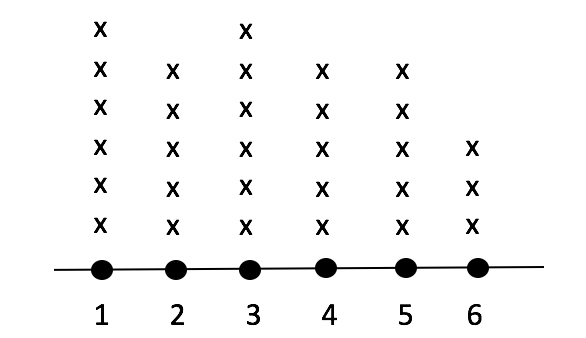# SSAT Middle Level Math : How to find the answer from a table

## Example Questions

1 3 Next →

### Example Question #21 : How To Find The Answer From A Table

Mrs. Smith’s class made a line plot to show the amount of sugar that each student consumes in a given day.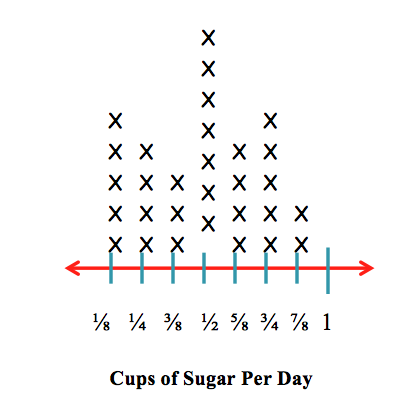How many students consumecup of sugar?Explanation:

Each X represents one person. There areXs above thetick mark on the line plot.

### Example Question #21 : Tables

Mrs. Smith’s class made a line plot to show the amount of sugar that each student has in a given day.How many students have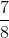cups of sugar?Explanation:

Each X represents one person. There areXs above thetick mark on the line plot.

### Example Question #212 : Data Analysis And Probability

Mrs. Smith’s class made a line plot to show the amount of sugar that each student has in a given day.How many students haveorcups of sugar?Explanation:

To solve this problem, we have to add the number of Xs above thetick mark to the number of Xs above thetick mark.

There areXs above thetick mark andXs above thetick mark.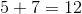### Example Question #213 : Data Analysis And Probability

Mrs. Smith’s class made a line plot to show the amount of sugar that each student has in a given day.How many students have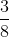of a cup of sugar?Explanation:

Each X represents one person. There areXs above thetick mark on the line plot.

### Example Question #21 : Tables

Mrs. Smith’s class made a line plot to show the amount of sugar that each student has in a given day.How many students have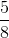of a cup of sugar?Explanation:

Each X represents one person. There areXs above thetick mark on the line plot.

### Example Question #21 : Tables

Find: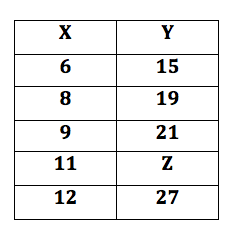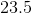Explanation:

If you are faced with a chart and a missing number, you need to figure out the pattern in the pairs that are shown.

If you think you have discovered the pattern, make sure to test it on each known pair and test if it is true for every pair.

The pattern will always stay the same. For these set of numbers the formula is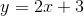.

Therefore,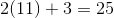.

### Example Question #214 : Data Analysis And Probability

The table below gives the number of students who scored in each of five  ranges on a standardized test.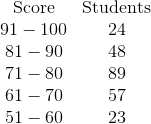No student scored below 51.

What percent of the students scored 71 or better (nearest whole number)?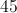%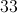%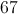%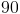%%

Explanation:

Add the numbers of students whose scores fell in the 71-80, 81-90, and 91-100 ranges: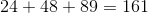Now add the number of students who took the test.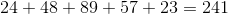To find what percent 161 is of 241, evaluate: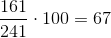67% of the students scored 71 or better.

### Example Question #11 : Make A Line Plot To Display A Data Set Of Measurements In Fractions And Solve Problems: Ccss.Math.Content.5.Md.B.2

The line chart shows how many books each student in Mrs. Smith's class read this week.  How many students are in Mrs. Smith's class?